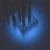0
Не ошибка

ODE's benchmark is slower with MCT

Michal Kvasnicka 11 месяцев назад • обновлен Pavel Holoborodko 11 месяцев назад

Hello, I just found strange behavior (bug?) of MATLAB R2018a (update 3) with latest version of MCT on the Path (Ubuntu Linux 16.04, 64bit).

If I run the bench() function with MCT on the path I get the following results:

>> bench(5)

ans =

0.0743    0.1146    0.0462    0.0832    0.4225    0.5393
0.0750    0.1108    0.0462    0.0845    0.3682    0.4330
0.0739    0.1176    0.0521    0.0895    0.4052    0.4539
0.0785    0.1275    0.0473    0.0826    0.3355    0.4691
0.0744    0.1155    0.0464    0.1066    0.3874    0.4228

The same benchmark run without MCT on the path produce the following results:

>> bench(5)

ans =

0.0745    0.1184    0.0130    0.0839    0.4043    0.5116
0.0798    0.1139    0.0133    0.0834    0.3796    0.4141
0.0809    0.1275    0.0141    0.1051    0.4728    0.4096
0.0850    0.1182    0.0132    0.0839    0.3576    0.4142
0.0884    0.1132    0.0134    0.0859    0.4168    0.4460

As you can see, the 3rd column (corresponding to the ODE's part of MATLAB benchmark) shows significantly (~4x) slower runs in a case of MCT on the path.

Could you clarify and/or reproduce this situation, please?

ОтветОтвет
Не ошибка

Hello Michal,

Toolbox overloads standard MATLAB routines to create elementary arrays - zeros, ones, eye, etc.

This is needed to support 'mp' type as argument in such functions, e.g. eye(100,'mp').

If requested type is not 'mp' then toolbox dispatches the call to built-in MATLAB functions (see arrayCreationOverload.m  for more details). Dispatch is quick but I expect it to be slower than direct call to double-precision functions.

MATLAB picks up toolbox overload when it is on the path.

Probably this is what causing slow-down in your tests.Ответ
Не ошибка

Hello Michal,

Toolbox overloads standard MATLAB routines to create elementary arrays - zeros, ones, eye, etc.

This is needed to support 'mp' type as argument in such functions, e.g. eye(100,'mp').

If requested type is not 'mp' then toolbox dispatches the call to built-in MATLAB functions (see arrayCreationOverload.m  for more details). Dispatch is quick but I expect it to be slower than direct call to double-precision functions.

MATLAB picks up toolbox overload when it is on the path.

Probably this is what causing slow-down in your tests.I am afraid that you are not right in this case. Please extract from standard matlab function bench.m the following content to the file bench_ode.m

--------------------------bench_ode.m-------------------------------------

function [t, n] = bench_ode
% ODE. van der Pol equation, mu = 1
F = @vanderpol;
y0 = [2; 0];
tspan = [0 eps];
[s,y] = ode45(F,tspan,y0);  %#ok Used  to preallocate s and  y
tspan = [0 450];
n = tspan(end);
tic
[s,y] = ode45(F,tspan,y0); %#ok Results not used -- strictly for timing
t = toc;
end

function dydt = vanderpol(~,y)
%VANDERPOL  Evaluate the van der Pol ODEs for mu = 1
dydt = [y(2); (1-y(1)^2)*y(2)-y(1)];
end

-----------------------------------------------------------------------------------

In a case of MCT on the path:

>> time = bench_ode

time =

0.0775

but in a case without MCT on the path:

>> time = bench_ode

time =

0.0238

MCT has some slowdown impact on ODE's functionality in general ... This is not acceptable behavior of presence MCT on path! So, I think that this behavior is a bug.The ode45 is m-script, it creates a lot of elementary arrays inside - which are dispatched through toolbox overloads (when it is on the path). That is why ode45 is slower.

Run ode45 through performance profiler and check where bottleneck is.

In general toolbox treats ODEs the same way as all other functionality - toolbox doesn't do any intrusive actions (e.g. replace standard scripts or else). MATLAB just picks up its overloads when it is on the path.I did not observed this problem with any other matlab algorithms (linear algebra, FFT, statistics, etc.). Degradation of ODE's algorithm is so significant (benchmark problem is only very simple example to show this problem in natura), that  is really not acceptable.

Finally, to avoid this problem, you propose only remove MCT from the path? Am I right?  But this is off/on solution. In a case of active MCT user will be always suffered by significant ODE's slowdown.@"I did not observed this problem with any other matlab algorithms (linear algebra, FFT, statistics, etc.)."

All of these in MATLAB are implemented directly in compiled languages C++/FORTRAN - that is why there is no difference.

On the contrary, ODE-related functionality is implemented in M-language with all the consequences for speed.

@"Finally, to avoid this problem, you propose only remove MCT from the path? Am I right?"

I do not create MATLAB's design rules and constraints. I just follow their rules as we all do.

I would be happy if someone would enlighten me with better workarounds.

Initially MATLAB was designed as procedural language, and OOP constructs were imposed later on to catch with modern trends. As a result, OOP support in MATLAB is very clumsy, slow and not flexible.

Сервис поддержки клиентов работает на платформе UserEcho